# Help please: A Modular Function: You must rewrite the code to use a modular function that...

A Modular Function:

You must rewrite the code to use a modular function that can be used again by other modules.

Your main program will call the function and pass the variables by value. Your function will take the appropriate action and return the required value . (Hint you will use additional variables in your revised code).

The called function must be able to accept the data passed to it by the function doing the calling. Only after the called function successfully receives the data can the data be manipulated to produce a useful result. An example of passing data between functions is provided in Program 6.1 in your book, which calls a function findMax().

Code to re-write:

#include <iostream>

using namespace std;

int main()

{

int count;

double num, total;

total = 0;

cout << "Please enter a number";

cin >> num;

for (count = 1; count <=num; count = count + 1)

{

// Re-write this section as a function

total = total + count;

cout << "Current number: " << count << endl;

cout << "Total so far: " << total << endl;

}

cout << "Your total is: " << total << endl;

return 0;

}

#include <iostream>

using namespace std;

int dummy(int num,int total);

int main()

{

int count;

double num, total;

total = 0;

cout << "Please enter a number";

cin >> num;

dummy(num,total);

cout << "Your total is: " << total << endl;

return 0;

}

int dummy(int num,int total){

int count;

for (count = 1; count <=num; count = count + 1)

{

// Re-write this section as a function

total = total + count;

cout << "Current number: " << count << endl;

cout << "Total so far: " << total << endl;

}

}

#### Earn Coin

Coins can be redeemed for fabulous gifts.

Similar Homework Help Questions
• ### In PEP8 code. assembly pep8 code. 30. Write an assembly language program that corresponds to the...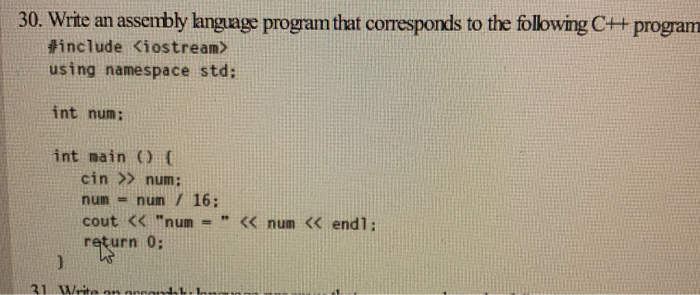In PEP8 code. assembly pep8 code. 30. Write an assembly language program that corresponds to the following C+ program #include <iostream> using namespace std; int num; int main() cin >> num: num = num/ 16; cout << "num = " << num << endl; return 0; 21 de

• ### Bly language program that c l orresponds to the following Cr+ program.Include the memory addr Wri...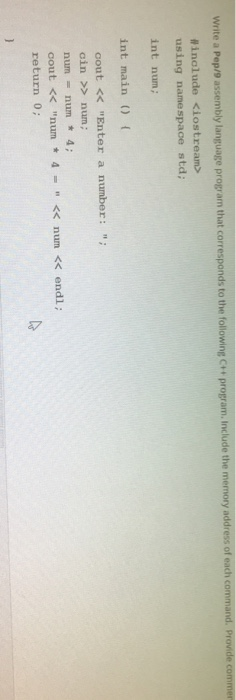bly language program that c l orresponds to the following Cr+ program.Include the memory addr Write a Pep/9 a include <iostream> using namespace std; int num; int main ( cout << "Enter a number:" cin >> num ; num = num * 4; cout << "num 4-<< num << endl; return 0 bly language program that c l orresponds to the following Cr+ program.Include the memory addr Write a Pep/9 a include using namespace std; int num; int main (...

• ### 12) 8 pts. Find the errors in the following code fragment and correct them. #i nclude...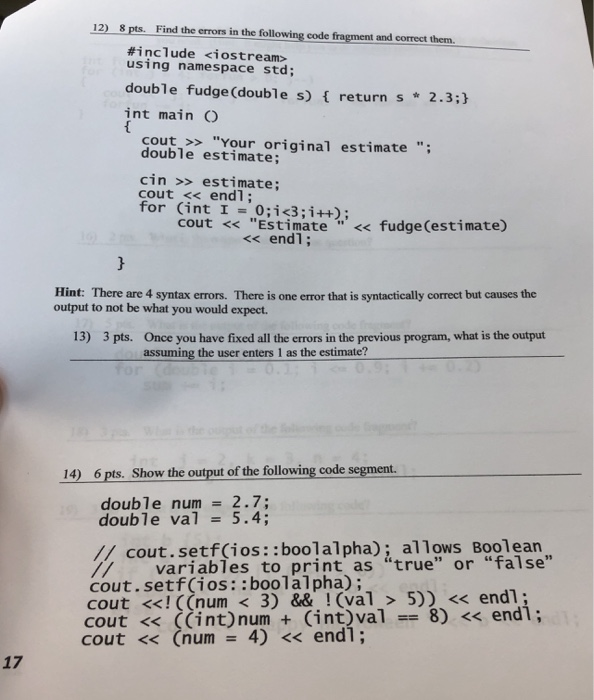12) 8 pts. Find the errors in the following code fragment and correct them. #i nclude <iostream> using namespace std; double fudge (double s) f return s 2.3; int mainO cout >> "Your original estimate" double estimate; cin >> estimate; cout << endl; for (int 1 = 0;1c3;i++); cout << "EStimate' < fudge(estimate) <<endl Hint: There are 4 syntax errors. There is one error that is syntactically correct but causes the output to not be what you would expect. Once...

• ### Need a FLOW Chart for that code. #include <iostream > using namespace std; int PowerFive(int); //Function...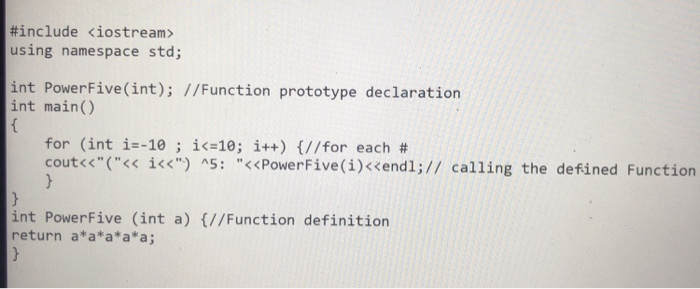Need a FLOW Chart for that code. #include <iostream > using namespace std; int PowerFive(int); //Function prototype declaration int main() for (int i=-10 ; i(z10; 1++) {//for each # cout<<"("<< ǐ<<") ^5. "<<PowerFive(1)くくendl;// calling the defined Function int PowerFive (int a) //Function definition return a*a*a*a*a;

• ### C++ Object Oriented assignment Can you please check the program written below if it has appropriately...

C++ Object Oriented assignment Can you please check the program written below if it has appropriately fulfilled the instructions provided below. Please do the necessary change that this program may need. I am expecting to get a full credit for this assignment so put your effort to correct and help the program have the most efficient algorithm within the scope of the instruction given. INSTRUCTIONS Create a fraction class and add your Name to the name fraction and use this...

• ### Convert the below code into if else selection: #include <iostream> using namespace std; int main() {...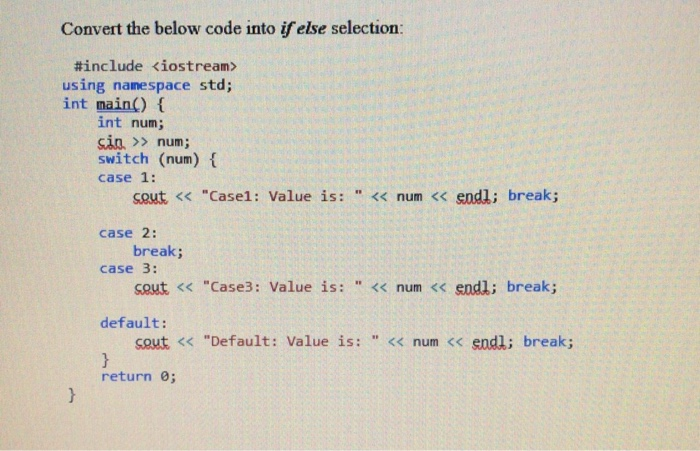Convert the below code into if else selection: #include <iostream> using namespace std; int main() { int num; sin. >> num; switch (num) { case 1: cout << "Casel: Value is: << num << endl; break; case 2: break; case 3: cout << "Case3: Value is: " << num << endl; break; default: cout << "Default: Value is: << num << endl; break; } return; }

• ### Question 19 4 pts Using the program below, please complete the program by adding 2 functions...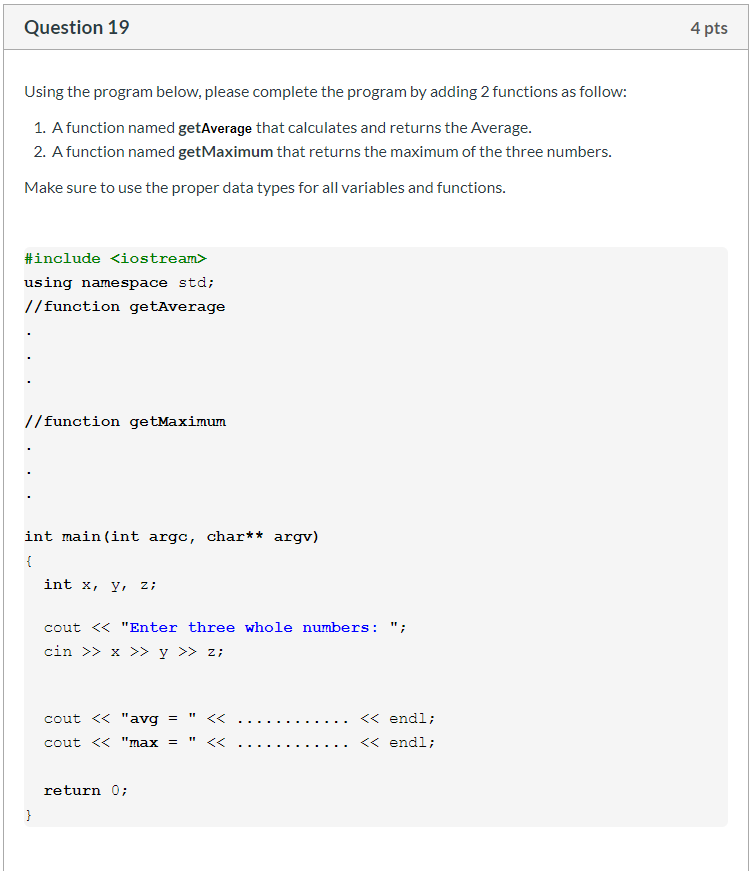Question 19 4 pts Using the program below, please complete the program by adding 2 functions as follow: 1. A function named getAverage that calculates and returns the Average. 2. A function named getMaximum that returns the maximum of the three numbers. Make sure to use the proper data types for all variables and functions. #include <iostream> using namespace std; //function getAverage //function getMaximum int main(int argc, char** argv) { int x, y, z; cout << "Enter three whole numbers:...

• ### 6. (Short answer) The C++ code below is very close to working, but, as written, won't...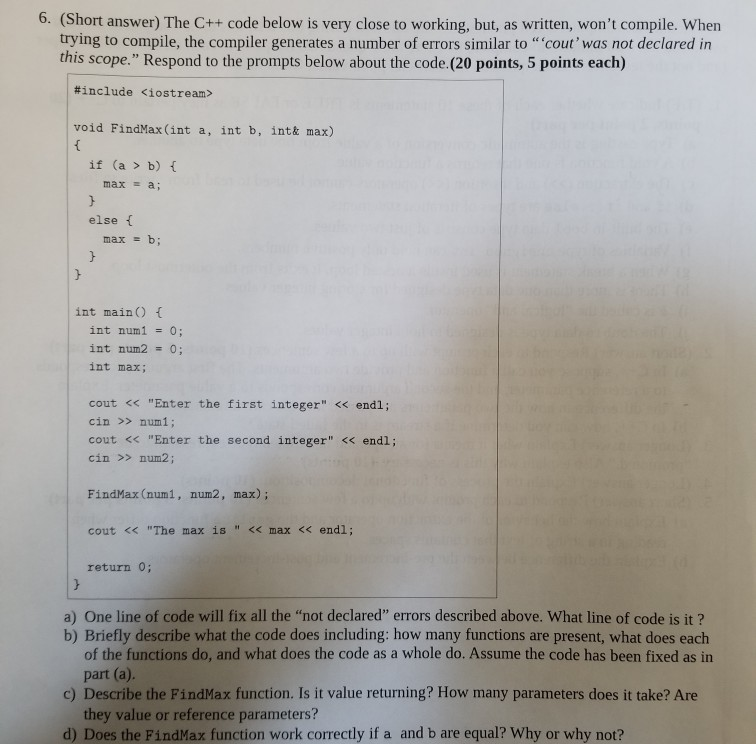6. (Short answer) The C++ code below is very close to working, but, as written, won't compile. When trying to compile, the compiler generates a number of errors similar to "cout' was not declared in this scope." Respond to the prompts below about the code.(20 points, 5 points each) #include <iostream> void FindMax(int a, int b, int& max) { if (a > b) max = a; else { max = b; } int main() { int num1 = 0; int...

• ### #include <iostream> using namespace std; bool binarySearch(int arr[], int start, int end, int target){ //your code...

#include <iostream> using namespace std; bool binarySearch(int arr[], int start, int end, int target){ //your code here } void fill(int arr[], int count){ for(int i = 0; i < count; i++){ cout << "Enter number: "; cin >> arr[i]; } } void display(int arr[], int count){ for(int i = 0; i < count; i++){ cout << arr[i] << endl; } } int main() { cout << "How many items: "; int count; cin >> count; int * arr = new...

In this experiment you will investigate the use of pointers and references in a C++ program.Enter, save, compile and execute the following program in MSVS. Call the new project “PtrsAndRefsExp1” and the program “ptrsAndrefs1.cpp”. Answer the questionsbelow:#include <iostream>using namespace std;int main(){int i;int *p;cout<<&i<<endl;cout<<p<<endl;cout<<&p<<endl<<endl;i=90;p = &i;cout<<i<<endl;cout<<(*p)<<endl;return 0;}Question 1: Please discuss the output (if any), and any errors or warnings your compiler gives. (Hint: Describe how the different operators are being used in differentstatements. Be as complete as possible in your description.)Step...